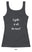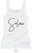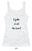"].join(""),l.id=h,(m?l:n).innerHTML+=f,n.appendChild(l),m||(n.style.background="",n.style.overflow="hidden",k=g.style.overflow,g.style.overflow="hidden",g.appendChild(n)),i=c(l,a),m?l.parentNode.removeChild(l):(n.parentNode.removeChild(n),g.style.overflow=k),!!i},x={}.hasOwnProperty,y;!B(x,"undefined")&&!B(x.call,"undefined")?y=function(a,b){return x.call(a,b)}:y=function(a,b){return b in a&&B(a.constructor.prototype[b],"undefined")},Function.prototype.bind||(Function.prototype.bind=function(b){var c=this;if(typeof c!="function")throw new TypeError;var d=u.call(arguments,1),e=function(){if(this instanceof e){var a=function(){};a.prototype=c.prototype;var f=new a,g=c.apply(f,d.concat(u.call(arguments)));return Object(g)===g?g:f}return c.apply(b,d.concat(u.call(arguments)))};return e}),q.touch=function(){var c;return"ontouchstart"in a||a.DocumentTouch&&b instanceof DocumentTouch?c=!0:w(["@media (",m.join("touch-enabled),("),h,")","{#modernizr{top:9px;position:absolute}}"].join(""),function(a){c=a.offsetTop===9}),c},q.csstransforms=function(){return!!F("transform")},q.csstransforms3d=function(){var a=!!F("perspective");return a&&"webkitPerspective"in g.style&&w("@media (transform-3d),(-webkit-transform-3d){#modernizr{left:9px;position:absolute;height:3px;}}",function(b,c){a=b.offsetLeft===9&&b.offsetHeight===3}),a},q.fontface=function(){var a;return w('@font-face {font-family:"font";src:url("https://")}',function(c,d){var e=b.getElementById("smodernizr"),f=e.sheet||e.styleSheet,g=f?f.cssRules&&f.cssRules?f.cssRules.cssText:f.cssText||"":"";a=/src/i.test(g)&&g.indexOf(d.split(" "))===0}),a};for(var G in q)y(q,G)&&(v=G.toLowerCase(),e[v]=q[G](),t.push((e[v]?"":"no-")+v));return e.addTest=function(a,b){if(typeof a=="object")for(var d in a)y(a,d)&&e.addTest(d,a[d]);else{a=a.toLowerCase();if(e[a]!==c)return e;b=typeof b=="function"?b():b,typeof f!="undefined"&&f&&(g.className+=" supports-"+(b?"":"no-")+a),e[a]=b}return e},z(""),i=k=null,e._version=d,e._prefixes=m,e._domPrefixes=p,e._cssomPrefixes=o,e.testProp=function(a){return D([a])},e.testAllProps=F,e.testStyles=w,g.className=g.className.replace(/(^|\s)no-js(\s|\$)/,"\$1\$2")+(f?" supports-js supports-"+t.join(" supports-"):""),e}(this,this.document); /*! * enquire.js v2.1.2 - Awesome Media Queries in JavaScript * Copyright (c) 2014 Nick Williams - http://wicky.nillia.ms/enquire.js * License: MIT (http://www.opensource.org/licenses/mit-license.php) */ !function(a,b,c){var d=window.matchMedia;"undefined"!=typeof module&&module.exports?module.exports=c(d):"function"==typeof define&&define.amd?define(function(){return b[a]=c(d)}):b[a]=c(d)}("enquire",this,function(a){"use strict";function b(a,b){var c,d=0,e=a.length;for(d;e>d&&(c=b(a[d],d),c!==!1);d++);}function c(a){return"[object Array]"===Object.prototype.toString.apply(a)}function d(a){return"function"==typeof a}function e(a){this.options=a,!a.deferSetup&&this.setup()}function f(b,c){this.query=b,this.isUnconditional=c,this.handlers=[],this.mql=a(b);var d=this;this.listener=function(a){d.mql=a,d.assess()},this.mql.addListener(this.listener)}function g(){if(!a)throw new Error("matchMedia not present, legacy browsers require a polyfill");this.queries={},this.browserIsIncapable=!a("only all").matches}return e.prototype={setup:function(){this.options.setup&&this.options.setup(),this.initialised=!0},on:function(){!this.initialised&&this.setup(),this.options.match&&this.options.match()},off:function(){this.options.unmatch&&this.options.unmatch()},destroy:function(){this.options.destroy?this.options.destroy():this.off()},equals:function(a){return this.options===a||this.options.match===a}},f.prototype={addHandler:function(a){var b=new e(a);this.handlers.push(b),this.matches()&&b.on()},removeHandler:function(a){var c=this.handlers;b(c,function(b,d){return b.equals(a)?(b.destroy(),!c.splice(d,1)):void 0})},matches:function(){return this.mql.matches||this.isUnconditional},clear:function(){b(this.handlers,function(a){a.destroy()}),this.mql.removeListener(this.listener),this.handlers.length=0},assess:function(){var a=this.matches()?"on":"off";b(this.handlers,function(b){b[a]()})}},g.prototype={register:function(a,e,g){var h=this.queries,i=g&&this.browserIsIncapable;return h[a]||(h[a]=new f(a,i)),d(e)&&(e={match:e}),c(e)||(e=[e]),b(e,function(b){d(b)&&(b={match:b}),h[a].addHandler(b)}),this},unregister:function(a,b){var c=this.queries[a];return c&&(b?c.removeHandler(b):(c.clear(),delete this.queries[a])),this}},new g}); /*! * imagesLoaded PACKAGED v4.1.0 * JavaScript is all like "You images are done yet or what?" * MIT License */ !function(t,e){"function"==typeof define&&define.amd?define("ev-emitter/ev-emitter",e):"object"==typeof module&&module.exports?module.exports=e():t.EvEmitter=e()}(this,function(){function t(){}var e=t.prototype;return e.on=function(t,e){if(t&&e){var i=this._events=this._events||{},n=i[t]=i[t]||[];return-1==n.indexOf(e)&&n.push(e),this}},e.once=function(t,e){if(t&&e){this.on(t,e);var i=this._onceEvents=this._onceEvents||{},n=i[t]=i[t]||[];return n[e]=!0,this}},e.off=function(t,e){var i=this._events&&this._events[t];if(i&&i.length){var n=i.indexOf(e);return-1!=n&&i.splice(n,1),this}},e.emitEvent=function(t,e){var i=this._events&&this._events[t];if(i&&i.length){var n=0,o=i[n];e=e||[];for(var r=this._onceEvents&&this._onceEvents[t];o;){var s=r&&r[o];s&&(this.off(t,o),delete r[o]),o.apply(this,e),n+=s?0:1,o=i[n]}return this}},t}),function(t,e){"use strict";"function"==typeof define&&define.amd?define(["ev-emitter/ev-emitter"],function(i){return e(t,i)}):"object"==typeof module&&module.exports?module.exports=e(t,require("ev-emitter")):t.imagesLoaded=e(t,t.EvEmitter)}(window,function(t,e){function i(t,e){for(var i in e)t[i]=e[i];return t}function n(t){var e=[];if(Array.isArray(t))e=t;else if("number"==typeof t.length)for(var i=0;i
Shopping Cart# I Fake it all the Time

## \$17.00

Fitted long tank with Solrae logo on the back front and text on the back, “I fake it all the time!”

Available in white and dark gray.

Women’s Spandex Jersey Tank

• 4.3 oz., 57/38/5 combed ringspun cotton/polyester/spandex, 40 singles
• Fabric laundered
• Self-fabric neck binding
• Longer body length, figure hugging silhouette
• Scoop front and back neckline
• Side seams

No reviews yet
0%
(0)
0%
(0)
0%
(0)
0%
(0)
0%
(0)